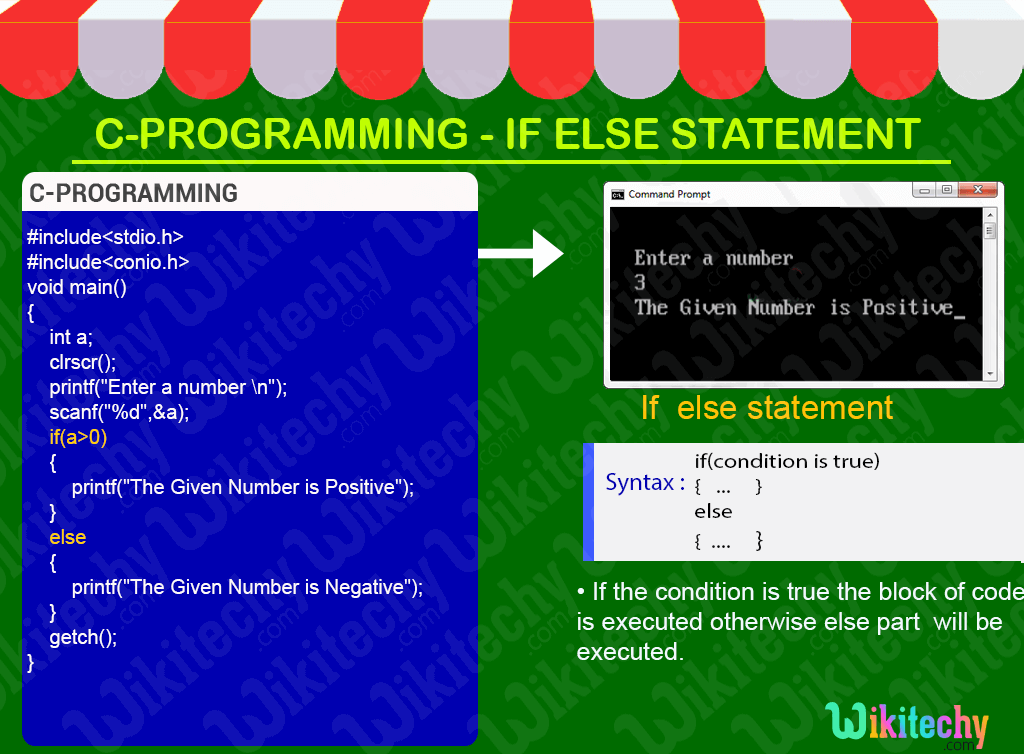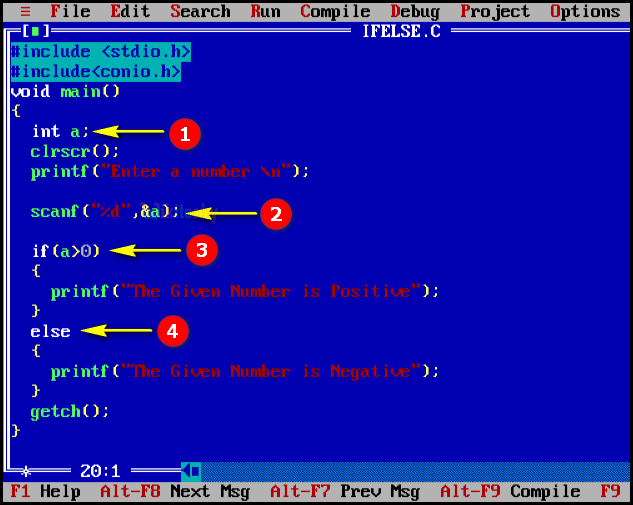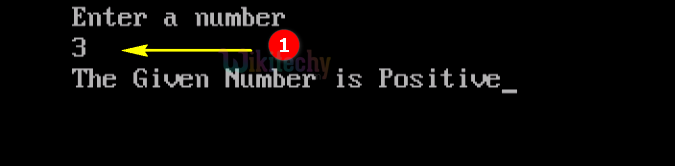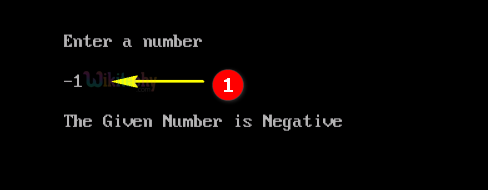# C - If Else StatementLearn C - C tutorial - C if else statement - C examples - C programs

## C If Else Statement- Definition and Usage

• In these type of statements, group of statements are executed when condition is true.
• If condition is false, then else part statements will be executed.## C Code - Explanation :1. Here we declare the variable “a” as integer .
2. In this statement we get the value of the variable “a” using scanf statement.
3. In this statement we check the condition that “a” value is greater than “0”, if condition satisfies it prints “The Given Number is Positive”.
4. Here “else statement” works which prints “The Given Number is Negative”.

## Sample Output - Programming Examples1. Here in this output we enter a value of the variable a= 3 so the given number is greater than “0” so the “if” section will be executed by displaying the output as “The Given Number is Positive”.1. Here in this output we enter a value of the variable a=-1 so the given number is less than “0” so the “else” section will be executed by displaying the output as “The Given Number is Negative”.